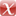# Time- and Space-Optimal Discrete Clock Synchronization in the Beeping Model

M. Feldmann, A. Khazraei, C. Scheideler, in: Proceedings of the 32nd ACM Symposium on Parallelism in Algorithms and Architectures (SPAA), ACM, 2020.

Conference Paper | English
Department
Abstract
We consider the clock synchronization problem in the (discrete) beeping model: Given a network of $n$ nodes with each node having a clock value $\delta(v) \in \{0,\ldots T-1\}$, the goal is to synchronize the clock values of all nodes such that they have the same value in any round. As is standard in clock synchronization, we assume \emph{arbitrary activations} for all nodes, i.e., the nodes start their protocol at an arbitrary round (not limited to $\{0,\ldots,T-1\}$). We give an asymptotically optimal algorithm that runs in $4D + \Bigl\lfloor \frac{D}{\lfloor T/4 \rfloor} \Bigr \rfloor \cdot (T \mod 4) = O(D)$ rounds, where $D$ is the diameter of the network. Once all nodes are in sync, they beep at the same round every $T$ rounds. The algorithm drastically improves on the $O(T D)$-bound of \cite{firefly_sync} (where $T$ is required to be at least $4n$, so the bound is no better than $O(nD)$). Our algorithm is very simple as nodes only have to maintain $3$ bits in addition to the $\lceil \log T \rceil$ bits needed to maintain the clock. Furthermore we investigate the complexity of \emph{self-stabilizing} solutions for the clock synchronization problem: We first show lower bounds of $\Omega(\max\{T,n\})$ rounds on the runtime and $\Omega(\log(\max\{T,n\}))$ bits of memory required for any such protocol. Afterwards we present a protocol that runs in $O(\max\{T,n\})$ rounds using at most $O(\log(\max\{T,n\}))$ bits at each node, which is asymptotically optimal with regards to both, runtime and memory requirements.
Publishing Year
Proceedings Title
Proceedings of the 32nd ACM Symposium on Parallelism in Algorithms and Architectures (SPAA)
LibreCat-ID

### Cite this

Feldmann M, Khazraei A, Scheideler C. Time- and Space-Optimal Discrete Clock Synchronization in the Beeping Model. In: Proceedings of the 32nd ACM Symposium on Parallelism in Algorithms and Architectures (SPAA). ACM; 2020. doi:10.1145/3350755.3400246
Feldmann, M., Khazraei, A., & Scheideler, C. (2020). Time- and Space-Optimal Discrete Clock Synchronization in the Beeping Model. In Proceedings of the 32nd ACM Symposium on Parallelism in Algorithms and Architectures (SPAA). ACM. https://doi.org/10.1145/3350755.3400246
@inproceedings{Feldmann_Khazraei_Scheideler_2020, title={Time- and Space-Optimal Discrete Clock Synchronization in the Beeping Model}, DOI={10.1145/3350755.3400246}, booktitle={Proceedings of the 32nd ACM Symposium on Parallelism in Algorithms and Architectures (SPAA)}, publisher={ACM}, author={Feldmann, Michael and Khazraei, Ardalan and Scheideler, Christian}, year={2020} }
Feldmann, Michael, Ardalan Khazraei, and Christian Scheideler. “Time- and Space-Optimal Discrete Clock Synchronization in the Beeping Model.” In Proceedings of the 32nd ACM Symposium on Parallelism in Algorithms and Architectures (SPAA). ACM, 2020. https://doi.org/10.1145/3350755.3400246.
M. Feldmann, A. Khazraei, and C. Scheideler, “Time- and Space-Optimal Discrete Clock Synchronization in the Beeping Model,” in Proceedings of the 32nd ACM Symposium on Parallelism in Algorithms and Architectures (SPAA), 2020.
Feldmann, Michael, et al. “Time- and Space-Optimal Discrete Clock Synchronization in the Beeping Model.” Proceedings of the 32nd ACM Symposium on Parallelism in Algorithms and Architectures (SPAA), ACM, 2020, doi:10.1145/3350755.3400246.

### Export

Marked Publications

Open Data LibreCat

### SourcesarXiv 2005.07388